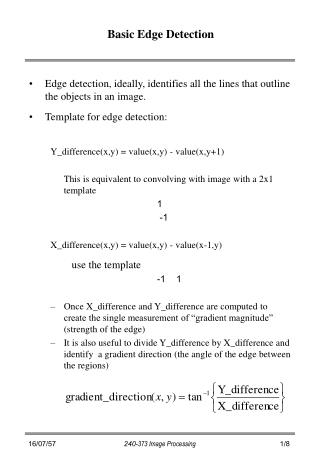Download PresentationBasic Edge Detection

# Basic Edge Detection - PowerPoint PPT PresentationDownload Presentation## Basic Edge Detection

- - - - - - - - - - - - - - - - - - - - - - - - - - - E N D - - - - - - - - - - - - - - - - - - - - - - - - - - -
##### Presentation Transcript

1. Basic Edge Detection • Edge detection, ideally, identifies all the lines that outline the objects in an image. • Template for edge detection: Y_difference(x,y) = value(x,y) - value(x,y+1) This is equivalent to convolving with image with a 2x1 template 1 -1 X_difference(x,y) = value(x,y) - value(x-1,y) use the template -1 1 • Once X_difference and Y_difference are computed to create the single measurement of “gradient magnitude” (strength of the edge) • It is also useful to divide Y_difference by X_difference and identify a gradient direction (the angle of the edge between the regions) 240-373 Image Processing

2. Example Image X_difference Y_difference Gradient Direction 1 1 1 1 1 0 0 0 0 * 0 0 0 0 0 * * * * * 1 1 1 1 1 0 0 0 0 * 0 0 0 1 1 * * * 1 1 1 0 0 0 0 -1 0 * 0 0 1 0 0 * * * * 1 1 0 0 0 0 -1 0 0 * 0 0 0 0 0 * * * * 1 1 0 0 0 0 -1 0 0 * * * * * * * * * * Technique 4: Sobel edge detection The Sobel 3 x 3 templates are normally given as X-direction Y-direction -1 0 1 1 2 1 -2 0 2 0 0 0 -1 0 1 -1 -2 -1 Example Image abs A + abs B Threshold at 12 240-373 Image Processing

3. 240-373 Image Processing

4. 240-373 Image Processing

5. 240-373 Image Processing

6. Second-order edge detection • An image such as • The basic Sobel vertical edge operator yields • Applying the edge operator again yields • This is similar to the differentiation operator applied to a straight line, e.g. if y = 3x - 2, then • Laplacian template • can reduce gradient of lighting • enhances noise 240-373 Image Processing

7. Pyramid Edge Detection Technique 5: Pyramid edge detection USE: To enhance substantial (strong and long) edges but to ignore the weak or short edges THEORY: • The image is cut down to quarter • Each pixel in the quarter-size image is an average of the four corresponding pixels in the full-size image • This repeats until unwanted edges are invisible • An edge detector is applied to the smallest image and where edge pixels have been found, an edge detector is applied to the corresponding four pixels in the next largest image OPERATION: • Create a second image of size m/2 x n/2 by evaluating for each 0 < i < mand 0< j < n • This is repeated and each generated image is kept • With the smallest image, perform some edge detection--such as Sobel • In pixels where edges are discovered, perform as edge detection operation on the group of four corresponding pixels in the next largest image • Continue to find the best edges down through the pyramid of images until the main edges in the original image have been discovered 240-373 Image Processing

8. Edge Following Technique 7: Simple edge following USE: Knowing that a pixel is on an edge, the edge will be followed so that an objected is outlined OPERATION: • Suppose that a position on the edge has been identified, call it (x,y) and flag this position as used • Evaluate all the 3x3 Sobel gradient values centered on each of the eight pixels surrounding (x,y) • Choose the three pixels with the greatest absolute gradient magnitude • Put these three pixel in a three-column array • Order them in row according to the gradient magnitude • Choose the one with greatest gradient magnitude • Now this pixel will be one of the direction 0-7 with respect to the pixel (x,y) given by the following map, where * is the position of pixel (x,y) 0 1 2 7 * 3 6 5 4 • Call the direction of travel d • Repeat the algorithm but look at only pixels in d, (d+1) mod 8, and (d-1) mod 8 directions • If no suitable high value of gradient magnitude is found, remove the pixel from the list and choose the next one of the three stored. Move up one row if all three are removed • Stop when the original pixel is reached, or execution has gone too long, or the number of rows in the list is very large 240-373 Image Processing